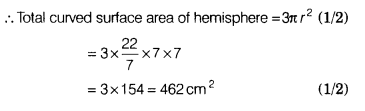# The radius of a solid hemisphere is 7 cm. Find its total curved surface area

The radius of a solid hemisphere is 7 cm. Find its total curved surface area.

Given, radius of solid hemisphere r = 7 cm